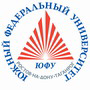Russian©  M. E. Abramyan (Southern Federal University, Shenzhen MSU-BIT University), 1998–2023

 Main Tasks Examples PT for MPI-2

 Overview Input-output operations Task groups Begin Integer Boolean If Case For While Series Proc Func Minmax Array Matrix String File Text Param Recur Dynamic Dynamic (obj) Tree Tree (obj)

# Selection statement

Case1. An integer in the range 1 to 7 is given. Output the name of the respective day of week: 1 — "Monday", 2 — "Tuesday", …, 7 — "Sunday".

Case2°. Given an integer K, output the respective examination mark: 1 — "bad", 2 — "unsatisfactory", 3 — "mediocre", 4 — "good", 5 — "excellent". If K is not in the range 1 to 5 then output string "error".

Case3. A number of month is given (as an integer in the range 1 to 12): 1 — January, 2 — February, etc. Output the name of the respective season: "Winter", "Spring", "Summer", "Autumn".

Case4°. A number of month is given (as an integer in the range 1 to 12): 1 — January, 2 — February, etc. Output the amount of days in the month for a non-leap year.

Case5. The arithmetic operations are numbered as: 1 — addition, 2 — subtraction, 3 — multiplication, 4 — division. The order number N of an operation and two real numbers A and B are given (N is an integer in the range 1 to 4, В is not equal to 0). Perform the operation with the operands A and B and output the result.

Case6. The units of length are numbered as: 1 — decimeter, 2 — kilometer, 3 — meter, 4 — millimeter, 5 — centimeter. The order number N of a unit of length and also the length L of a segment are given (N is an integer in the range 1 to 5, L is a real number). Output the length of the segment in meters.

Case7. The units of weight are numbered as: 1 — kilogram, 2 — milligram, 3 — gram, 4 — ton, 5 — centner (= 100 kilograms). The order number N of a unit of weight and the mass M of a solid are given (N is an integer in the range 1 to 5, M is a real number). Output the mass of the solid in kilograms.

Case8. Given two integers D (day) and M (month) representing a correct date of a non-leap year, output values D and M for the previous date.

Case9°. Given two integers D (day) and M (month) representing a correct date of a non-leap year, output values D and M for the next date.

Case10°. A robot can move in four directions ("N" — north, "W" — west, "S" — south, "E" — east) and perform three digital instructions: 0 — "move in the former direction", 1 — "turn left", −1 — "turn right". A symbol C (an initial direction of the robot) and an integer N (an instruction) are given. Output the direction of the robot (as symbol) after performing the instruction.

Case11. A locator can be focused on the directions "N" (north), "W" (west), "S" (south), "E" (east) and perform three digital instructions: 1 — "turn left", −1 — "turn right", 2 — "turn on 180°"). A symbol C (an initial direction of the locator) and two integers N1 and N2 (instructions) are given. Output the direction of the locator (as symbol) after performing the instructions.

Case12. Elements of a circle are numbered as: 1 — radius R, 2 — diameter D = 2·R, 3 — length L = 2·π·R of the circumference, 4 — area S = π·R2. The order number of one element and its value (as a real number) are given. Output values of other elements in the same order. Use 3.14 for a value of π.

Case13. Elements of a right isosceles triangle are numbered as: 1 — leg a, 2 — hypotenuse c = a·(2)1/2, 3 — altitude h drawn onto hypotenuse (h = c/2), 4 — area S = c·h/2. The order number of one element and its value (as a real number) are given. Output values of other elements in the same order.

Case14. Elements of an equilateral triangle are numbered as: 1 — side a, 2 — radius R1 of inscribed circle (R1 = a·(3)1/2/6), 3 — radius R2 of circumscribed circle (R2 = 2·R1), 4 — area S = a2·(3)1/2/4. The order number of one element and its value (as a real number) are given. Output values of other elements in the same order.

Case15. The suits of playing cards are numbered as: 1 — spades, 2 — clubs, 3 — diamonds, 4 — hearts. Card values "Jack", "Queen", "King", "Ace" are numbered as 11, 12, 13, 14 respectively. A card value N (as an integer in the range  6 to 14) and a suit M (as an integer in the range 1 to 4) are given. Output the card description as: "six of diamonds", "queen of spades", etc.

Case16. Given an age in years (as an integer in the range 20 to 69), output its alphabetic equivalent as: "twenty years", "thirty-two years", "forty-one years", etc.

Case17. Given an order number of some training task (as an integer in the range 10 to 40), output its alphabetic equivalent as: "the eighteenth task", "the twenty-third task", "the thirtieth task", etc.

Case18°. Given an integer in the range 100 to 999, output its alphabetic equivalent. For example, 100 — "one hundred", 256 — "two hundred and fifty-six", 814 — "eight hundred and fourteen", 901 — "nine hundred and one".

Case19. One of the Asian calendars uses 60-years periods divided into 12-years cycles, which are associated with a color: green, red, yellow, white, black. Each year in a cycle is connected with some animal: rat, cow, tiger, hare, dragon, snake, horse, sheep, monkey, hen, dog, pig. Given some year (as positive integer), output its name provided that 1984 is "The Green Rat`s year".

Case20. Given two integers D (day) and M (month) that represent a correct date, output the zodiacal name corresponding to this date: "Aquarius" 20.1–18.2, "Pisces" 19.2–20.3, "Aries" 21.3–19.4, "Taurus" 20.4–20.5, "Gemini" 21.5–21.6, "Cancer" 22.6–22.7, "Leo" 23.7–22.8, "Virgo" 23.8–22.9, "Libra" 23.9–22.10, "Scorpio" 23.10–22.11, "Sagittarius" 23.11–21.12, "Capricorn" 22.12–19.1.Designed byM. E. Abramyan and V. N. Braguilevsky Last revised:01.01.2023